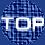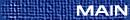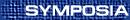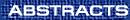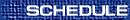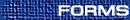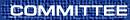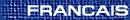Combinatorics / Combinatoire
(C. Chauvre, S. Corteel and P. Leroux, Organizers)

F. BERGERON, Université du Québec à Montréal, C.P. 8888, Succ. Centre-Ville, Montréal, Québec  H3C 3P8
Ideals of quasi-symmetric polynomials and related varieties

For many nice families of ideals generated by quasi-symmetric polynomials in n variables, x1¼xn, we give explicit description of their (reduced) Gröbner basis. This implies that we have explicit combinatorial descriptions for basis of the quotient of the ring of polynomials in x1¼xn by each of these ideals. The dimension (and Hilbert series) of these spaces are very nice from a combinatorial view point.

M. BOUSQUET, LaCIM, UQAM, Case Postale 8888, succursale Centre-ville, Montréal, Québec  H3C 3P8
Dénombrement de 2-arbres solides

Le but de ce travail est d'obtenir l'énumération des 2-arbres solides selon le nombre d'arêtes (ou de triangles) ainsi que selon la distribution des degrés des arêtes. Nous obtenons d'abord le dénombrement des 2-arbres solides orientés en utilisant les méthodes de la théorie des espèces. Pour obtenir le dénombrement des 2-arbres solides non orientés, nous utilisons la notion d'espèce quotient qui provient d'une spécialisation de la théorie de Pólya.

The main goal of thiswoorkr is to enumerate solid 2-trees according to the number of edges (or triangles) and also according to the edge degree distribution. We first enumerate oriented solid 2-trees using the general methods of the theory of species. In order to obtain non oriented enumeration formulas we use quotient species which consists in a specialization of Pólya theory.

M. BOUSQUET-MELOU, CNRS, Université Bordeaux 1, Talence Cedex, France
Walks in a quarter plane

We study planar walks that start from a given point (i0,j0), take their steps in a finite set \frak S, and are confined in the first quadrant x ³ 0, y ³ 0. Their enumeration can be attacked in a systematic way: the generating function Q(x,y;t) that counts them by their length (variable t) and the coordinates of their endpoint (variables x,y) satisfies a linear functional equation encoding the step-by-step description of walks. For instance, for the square lattice walks starting from the origin, this equation reads

 æè xy -t(x+y+x2y+xy2) öø Q(x,y;t)=xy-xtQ(x,0;t)-ytQ(0,y;t).
The central question addressed in this talk is the nature of the series Q(x,y;t). When is it algebraic? When is it D-finite (or holonomic)? Can these properties be derived from the functional equation itself?

Our first result is a new proof of an old theorem due to Kreweras, according to which one of these walk models has, for mysterious reasons, an algebraic generating function. Then, we provide a new proof of a holonomy criterion recently proved by M. Petkovsek and the author. Finally, we exhibit a simple model with a non-holonomic generating function (joint work with M. Petkovsek). In all cases, we work directly from the functional equation.

GERARD DUCHAMP, Rouen
Algèbres de Hopf de fonctions quasi-symétriques non commutatives / Hopf algebras of non commutative quasi-symmetric functions

On construit diverses algèbres de Hopf généralisant celles des fonctions symétriques et quasi-symtriques.

We contruct various Hopf Algebras generalizing the Hopf Algebras of Symmetric and Quasi-Symmetric Functions.

I. GESSEL, Brandeis University, Waltham, Massachusetts  02454, USA
Two-stack-sortable multipermutations

To ``stack-sort'' a permutation we represent the permutation as a binary decreasing tree, and then read the tree in postorder. A permutation is called two-stack-sortable if stack-sorting it twice yields an identity permutation.

Doron Zeilberger proved Julian West's conjecture that the number of two-stack-sortable permutations of {1,2,¼,n} is 2(3n)!/((n+1)! (2n+1)!). Bousquet-Mélou, Bóna, and Travis independently refined Zeilberger's result by showing that the number of two-stack-sortable permutations of {1,2,¼, i+j+1} with i descents is

 (2i+j+1)! (i+2j+1)!(i+1)! (2i+1)! (j+1)! (2j+1)! .

We consider a generalization of the stack-sorting operation in which binary decreasing trees are replaced by (r+1)-ary decreasing trees. These trees correspond to certain multiset permutations called ``r-permutations'', which reduce to ordinary permutations when r=1. We generalize the result of Bousquet-Mélou, Bóna, and Travis to r-permutations. We classify the descents of r-permutations into r types and show that the number of two-stack-sortable r-permutations with il descents of type l for l=1,¼,r is

 1n2 rÕ l=0 nn-il \binom2n-il-1il,
where n=1+i0+¼+ir.

This is joint work with Dapeng Xu.

IAN GOULDEN, University of Waterloo, Waterloo, Ontario  N2L 3G1
Hurwitz numbers and combinatorics

>From a geometric point of view, Hurwitz numbers count ramified covers of the sphere by a Riemann surface of specified genus, with branching above infinity specified by a specified partition, and simple branching above other prescribed points. From a combinatorial point of view, these are equivalent to a particular class of ordered factorizations in the symmetric group. We describe recent results and conjectures for Hurwitz numbers and generalizations that have been obtained from this combinatorial point of view.

A. GOUPIL, Université du Québec à Montréal, Montréal, Québec  H3C 3P8
Polynômes de classes de Sn / Class Polynomials of Sn

A chaque classe de conjugaison Cm du groupe sym\' etrique Sn, nous associons un polynôme symétrique wm(x) que nous appelons polynôme de classe. Lorsque les polynômes wm(x) `sont' valués sur les contenus d'un partage l de n, on obtient le caractère irréductible normalisé [(|Cm|)/(fl )]clm. Les polynômes de classes forment une nouvelle base de l'espace L des polynômes symétriques. De plus, le produit wm(x) wa(x) se décompose dans la base { wm(x)}m comme le produit de classes Cm *Cl se décompose dans la base {Cm}m du centre de l'algèbre C[S¥] du groupe symétrique infini. Nous utilisons les polynômes wm(x) pour mettre au point des formules énumératives pour le nombre de tableaux standards gauches f(l/m) et pour les nombres de Kostka Kl/m. De plus, nous construisons des q-analogues des polynômes wm(x) pour l' algèbre de Hecke du groupe symétrique.

To each conjugacy class Cm of the symmetric group Sn, we associate a symmetric polynomial wm(x) that we call class polynomial. When the polynomial wm(x) is evaluated on the content of a partition l of n, we obtain the normalized irreducible character [(|Cm|)/(fl )]clm. The set of class polynomials forms a new linear basis for the space L of symmetric polynomials. Furthermore, the product wm(x)wa(x) decomposes in the basis {wm(x)}m precisely as the product of classes Cm*Cl decomposes in the basis {Cm}m of the center of the algebra C[S¥] of the infinite symmetric group. We use the polynoms wm(x) to establish enumerative formulae for the number f(l/m) of skew standard tableaux and for the Kostka numbers Kl/m. We also construct q-analogs of the polynomials wm(x) for the Hecke algebra of Sn.

G. LABELLE, Université du Québec à Montréal, Montréal, Québec
Structures partiellement étiquetées asymétriques

Soit ZF la série indicatrice des cycles d'une espèce F. En conformité avec la théorie de Pólya, on a la formule ZF°G = ZF°ZG, même lorsque G(0) ¹ 0. Cependant, dans le cas de la série indicatrice d'asymétrie, GF, des exemples simples montrent que l'égalité n'a généralement pas lieu, c.-à-d., GF°G ¹ GF°GG, lorsque G(0) ¹ 0; bien qu'elle ait toujours lieu lorsque G(0)=0. Nous introduisons une variante, GF, x, de la série GF qui satisfait l'égalité GF°G = GF, x°GG, où x = G(0). Nous étudions le comportement de la série GF, x face aux opérations combinatoires, en présentons divers exemples explicites et les appliquons au dénombrement de quelques classes de structures partiellement étiquetées asymétriques.

P. LALONDE, LaCIM, UQAM
Partitions planes descendantes et dualité de chemins

Les partitions planes descendantes apparaissent dans les conjectures de Mills, Robbins et Rumsey : elles seraient en bijection avec matrices à signes alternants. Ces partitions forment un ensemble partiellement ordonné qui possède un unique anti-automorphisme, noté t. D après les conjectures, t se traduirait en une op'eration très simple sur les matrices à signes alternants : la réflexion suivant un axe vertical. Pourtant, la description directe de t dans le cadre des partitions planes descendantes est assez complexe.

On peut cependant utiliser la méthodologie de Gessel-Viennot pour coder ces partitions en configurations de chemins sans intersection. On retrouve alors une description simple de t : il s agit de la dualité de chemins, mise en évidence (dans d autres contextes) par ces mêmes auteurs.

V. LISKOVETS, Institute of Mathematics, National Academy of Sciences of Belarus, Minsk, 220072  Belarus
Some asymptotic distribution patterns for planar maps

We focus mainly on two different asymptotic cardinality patterns for planar maps. They are of the form CnbRn (as n, the number of edges, tends to infinity), where b=-5/2 or -3/2 and C and R > 1 are constants depending on the maps under consideration. The value -5/2 of the ``critical exponent'' b is known to be valid for a large family of classes of rooted maps such as arbitrary, non-separable, polyhedral, cubic and Eulerian maps. On the contrary, b=-3/2 for various classes of rooted outerplanar maps (including plane trees) as well as maps of some singular types. We retrieve both of these values as particular cases of a general unified pattern and present some evidence and some simple arguments that justify this approach. This idea is illustrated in more detail for two specific classes of maps: Eulerian and self-dual.

Another pattern to be discussed briefly concerns the tail distribution of vertices by valency. We also touch on counting the total number of vertices in rooted maps.

PETER MCNAMARA, MIT

MARNI MISHNA, LaCIM/UQAM

J. MORSE, University of Pennsylvania
k-Schur functions and Kostka coefficients

We introduce a family of symmetric functions called the k-Schur functions and show how these elements generalize the Schur functions. We then discuss how a new type of tableaux could lead to finding expansion coefficients for the Hall-Littlewood and Macdonald polynomials in terms of k-Schur functions.

I. PAK, MIT, Cambridge, Massachusetts, USA
Fighting the involution principle

About 20 years ago, Garsia and Milne introduced the involution principle, which quickly became a powerful tool for creating bijections out of existing analytic proofs. Despite its popularity, the resulting bijections often lack simplicity and transparency, in contrast with many ``traditional'' bijections. This is our first attempt to understand and explain this striking phenomenon.

In this talk we restrict ourselves to the well studied case of Andrews' partition identities, which generalize the classical Euler's identity (the number of partitions of n into odd parts is equal to the number of partitions of n into distinct parts.) These were later extended by Cohen and Remmel. The latter also found a bijective proof by the involution principle, which was studied further by O'Hara. We will generalize these identities even further, and present an algebraic approach to bijection in these cases.

F. RUSKEY, University of Victoria, Victoria, British Columbia
Counting aperiodic strings with given elementary symmetric function evaluations

For fixed k and values t[1¼k] we try to count the number of aperiodic strings a[1¼n] for which Sk(a) = tk, where Sk is the k-th elementary symmetric function. The alphabet of the string a is a ring R. After setting up a general framework, specific results are given for the following cases:

(a) R is Z(q), the ring of integers mod q, and k=1, or q=4 and k=2.
(b) R is F(q), the finite field of q elements, and k = 3.
Using (b), we are able to count the number of odd degree monic irreducible polynomials over F(q) whose next 3 leading coefficients are prespecified and whose degree is odd. The efficacy of our formulae will be stated in rops, the number of ring operations necessary to evaluate them. Part of the development involves a new multivariate form of Mobius inversion.

C. SAVAGE, North Carolina State University, Raleigh, North Carolina, USA
Anti-lecture hall compositions

In 1997, Bousquet-Mélou and Eriksson proved a surprising result, known as the Lecture Hall Theorem: The number of partitions l = (l1,¼,lk) of n satisfying

 l1k ³ l2k-1 ³ ¼ ³ lk-12 ³ lk1 ³ 0
is equal to the number of partitions of n into odd parts of size at most 2k-1. In this talk, we prove the Anti-lecture Hall Theorem, a corresponding result about the number of compositions l = (l1,¼,lk) of n satisfying

 l11 ³ l22 ³ ¼ ³ lkk ³ 0.
To our knowledge this is a new result that complements the family of the lecture hall theorems. This is joint work with Sylvie Corteel.

G. SCHAEFFER, Loria-CNRS, ampus Sciences, Vandoeuvre-les-Nancy
The diameter of random planar maps

A planar map is a proper embedding of a graph in the sphere, considered up to homeomorphisms of the sphere. Following the work of Tutte in the sixties, various families of planar maps (triangulations, quadrangulations, eulerian, etc.) have been (and still are) enumerated with respect to their number of edges, vertices (see Liskovets' talk here).

We shall consider the uniform distribution on quadrangulations with n faces, and view them as random discretised surfaces. Combining bijections and enumerative results with probabilistic ideas, we show that one can study distances in these random quadrangulations. In particular the diameter of a random quandrangulation with n faces behave like n(1/4).

R. STANLEY, MIT, Cambridge, Massachusetts, USA
Kerov's character polynomial and irreducible symmetric group of rectangular shape

Shortly before his untimely death in 2000 Sergei Kerov introduced in a lecture a polynomial Sk(R2,R3,...,Rk+1) which encodes the value cl(w) of any irreducible character cl of the symmetric group Sn (for any n) at a k-cycle w. For instance, S2=R3, S3=R4+R2, S4=R5+5R3, S5 = R6+15R4+5R22+8R2. This polynomial is not well-understood, e.g., it is not known whether its coefficients are nonnegative. The coefficients of the linear terms Ri, however, have a combinatorial interpretation, first obtained by P. Biane. There is an alternative proof based on a new formula for cl(w), for any w Î Spq, when l has p parts all equal to q. It is an interesting open problem to extend this result to more general l.

B. VAN RENSBURG, York University, Toronto, Ontario  M3J 1P3
Exchange relations, Dyck paths and copolymer adsorption

A certain model of directed copolymer adsorption is equivalent to the enumeration of Dyck paths. We exploit a type of symmetry (we call this an exchange relation) to solve a specific model of an infinite family of periodically coloured copolymers adsorbing in a wall. We also find an asymptotic expression for the critical adsorption point in these models as a function of the period of the colouring. This is collaborative work with Andrew Rechnitzer.

X. VIENNOT, LaBRI, Université Bordeaux I, 33405  Talence, France
Gravitation quantique Lorentzienne et empilements de dominos / Lorentzian quantum gravity and heaps of dimers

La théorie des empilements de pièces fut introduite par l'orateur en 1985 afin de donner une vision ``géométrique'' de la théorie algébrique des monoïdes de commutations développée par Cartier et Foata en 1969. Ces empilements de pièces permettent d'unifier ou d'interpréter diverses théories combinatoires (polynômes orthogonaux, polynômes chromatiques, etc).

Des interactions et applications ont été développées par l'école bordelaise vers la physique statistique: modèles des animaux dirigés, de gaz avec particules dures, le modèle SOS, polyominos et fonctions q-Bessel, etc...

Cet exposé a pour but de relater une nouvelle application des empilements de pièces vers un tout autre domaine de la physique thé orique: la gravitation quantique Lorentzienne en dimension 2. Cette application fut développée récemment par P. Di Francesco, E. Guitter, C. Kristjansen, faisant suite aux travaux de J. Ambjorn et R. Loll. L'espace temps est discrétisé par des triangulations dites Lorentziennes. Le modèle de physique revient à expliciter certaines sé ries génératrices relatives à ces objets combinatoires. La résolution du modèle donnée par ces physiciens provient de lemmes et bijections de base sur les empilements de pièces.

The theory of heaps of pieces was introduced by the author in 1985 in order to give a ``geometric'' version of the algebraic theory of commutation monoids developed by Cartier and Foata in 1969. These heaps of pieces unify and give interpretations of various combinatorial theories (orthogonal polynomials, chromatic polynomials, etc).

Interactions and applications with statistical mechanics have been developed by the Bordeaux school: directed animal model, gaz model with hard core interactions SOS model, polyominoes and q-Bessel functions, etc...

The purpose of this talk is to expose a new application of the theory of heaps of pieces to another chapter of theoretical physics: 2D Lorentzian quantum gravity. This application has been recently developed by P. Di Francesco, E. Guitter, C. Kristjansen, following work of J. Ambjorn and R. Loll. The space time is discretized by some triangulations called Lorentzian. The physics model is equivalent to give explicit expressions for certain generating functions related to these combinatorial objects. The solution of the model given by these physicists comes from some basic lemma and bijections about heaps of pieces.

T.R. WALSH, Department of Computer Science, UQÀM, Montreal, Quebec  H3C-3P8
Génération de chaque mot d'un code gray en temps constant / Constant time generation of each word of a gray code

Un mot de longueur n est une suite finie g,g,...,g[n] de lettres, qui sont des membres d'un ensemble fini, un alphabet. Un code Gray est une famille infinie L1, L2,..., où Ln est une liste de mots, tous de longueur n, telle que chaque mot sauf le premier dans chaque liste Ln est obtenue de son prédécesseur en changeant un nombre de lettres borné indépendamment de n. Il y a des codes Gray pour beaucoup d'objets combinatoires: chaînes binaires, combinaisons, permutations, compositions d'un entier, partages d'un ensemble, partages d'un entier, mots de Dyck et d'autres représentations des arbres binaires. Pour quelques-uns de ces codes Gray-chaînes binaires, combinaisons, permutations, compositions d'un entier et partages d'un ensemble-G. Ehrlich a trouvé des algorithmes pour engendrer chaque mot sauf le premier en temps borné indépendamment de la longueur des mots et sa mé thode a été appliquée par d'autres chercheurs aux autres codes Gray. Dans cette confér ence il est démontré que sa méthode marche pour tout code Gray tel que tous les mots dans une liste avec le même pré fixe g,g,...,g[i-1] forment un intervalle de mots consécutifs dans lequel la prochaine lettre g[i] atteint au moins deux valeurs distinctes. En plus, sa méthode est généralisée pour permettre g[i] de n'atteindre qu'une seule valeur si un seul mot a le préfixe g,g,...,g[i-1]-cette condition est satisfaite par la vaste majorité de codes Gray dans la littérature. Un nouveau code Gray est présenté pour les compositions d'un entier avec chaque partie bornée entre 0 et un entier positif et pour les permutations de {1,2,...,n} avec r inversions dont les vecteurs d'inversions sont les compositions de r avec n-1 parties bornées par n-1,n-2,...,2,1; la généralisation de la méthode d'Ehrlich permet la génération de ces objets en temps constant.

A length-n word is a finite chain g,g,...,g[n] of letters which are elements of a finite set, an alphabet. A Gray code is an infinite family L1, L2,..., where Ln is a list of length-n words such that every word except the first one in each list Ln is obtained from its predecessor by changing a number of letters bounded independently of n. There are Gray codes for many combinatorial objects: binary chains, combinations, permutations, integer compositions, set partitions, integer partitions, Dyck words and other representations of binary trees. For some of these Gray codes-binary chains, combinations, permutations, integer compositions and set partitions-G. Ehrlich found algorithms for generating each word except the first one in a time bounded independently of the word length and his method has been applied by other researchers to other Gray codes. In this talk we prove that his method works for any Gray code such that all the words in a list with the same prefix g,g,...,g[i-1] form an interval of consecutive words in which the next letter g[i] takes at least two distinct values. Furthermore, we generalize his method to allow g[i] to take only one value if only one word has the prefix g,g,...,g[i-1]-this condition is satisfied by the vast majority of Gray codes in the literature. We present a new Gray code for integer compositions with each part bounded between 0 and a positive integer and for the permutations of {1,2,...,n} with r inversions whose inversion vectors are the compositions of r with n-1 parts bounded by n-1,n-2,...,2,1; using the generalisation of Ehrlich's method we generate each of these objects in constant time.

J. WEST, University of Victoria, Victoria, British Columbia
Somos sequences and perfect matchings

Linear recurrences (and, with them, ordinary generating functions) are ubiquitous in combinatorics, as part of a broad general framework that is well-studied and well-understood; in contrast, bilinear recurrences such as sn+4 = (sn+1sn+3+sn+22)/sn are encountered far less often, and these encounters tend to be viewed in isolation from one another.

The bilinear recurrence relation quoted above is the Somos-4 recurrence, part of a general family of ën/2û-degree sequences. Although these have received a fair bit of attention in the past few years, until recently algebra remained one step ahead of combinatorics, leaving us temporarily in the unusual position of being able to enumerate combinatorial objects for which we lack a combinatorial description.

In this talk, we produce a family of graphs whose perfect matchings are enumerated by the Somos-4 sequence, and a second family whose perfect matchings are enumerated by Somos-5. The recurrences that govern these sequences are also special cases of the Gale-Robinson family of recurrences: S(n)=[S(n-i1)S(n-i2)+S(n-j1)S(n-j2)]/S(n-k), where k=i1+i2=j1+j2. The results for Somos-4 and Somos-5 thus give us some hope that a similar family of graphs can be constructed for any Gale-Robinson recurrence.

This is joint work with Jim Propp and Mireille Bousquet-Melou. Many of these results have also been obtained independently by Propp's team of undergraduate researchers at Harvard.

C. YAN, Texas A&M University, College Station, USA
Goncarov polynomials and parking functions

The Goncarov polynomials gn(x;a0,a1,¼,an-1) are polynomials defined by the biorthogonality relation:

 e(ai) Di gn(x;a0,a1,¼,an-1) = n! din,
where e(a) is evaluation at a. Goncarov polynomials form a natural basis for generalized parking functions. In particular, expanding xn as a linear combination of Goncarov polynomials leads to a decomposition of an arbitrary sequences of positive integers into two subsequences: a maximum parking function and a subsequence of higher values. From this combinatorial decomposition, we derive linear recursions for sum enumerators, expected sums of u-parking functions, and higher moments of sums of u-parking functions. These recursions yield explicit formulas for these quantities in terms of Goncarov polynomials.

DORON ZEILBERGER, Rutgers University, New Brunswick, New Jersey  08903, USA
Deranged Wilf classes

Aaron Robertson found a surprising and deep refinement of the classical enumerative results that the number of 132-avoiding permutations equals the number of 123-avoiding permutations. This is probably but the tip of a beautiful deranged theory of Wilf Classes.

J. ZENG, Institut Girard Desargues, Universite Lyon 1, 43, Bd du 11 Novembre 1918, 69622  Villeurbanne Cedex, France
New identities of Hall-Littlewood polynomials and new identities of Rogers-Ramanujan type

Starting from Macdonald's summation formula of Hall-Littlewood polynomials over bounded partitions and its even partition analogue, Stembridge (Trans. Amer. Math. Soc. (2) 319(1990), 469-498) derived sixteen multiple q-identities of Rogers-Ramanujan type. Inspired by our recent results on Schur functions (Adv. Appl. Math. 27(2001), 493-509) and based on computer experiments we obtain two further such summation formulae of Hall-Littlewood polynomials over bounded partitions and derive six new multiple q-identities of Rogers-Ramanujan type. This talk is base on joint works with F. Jouhet.